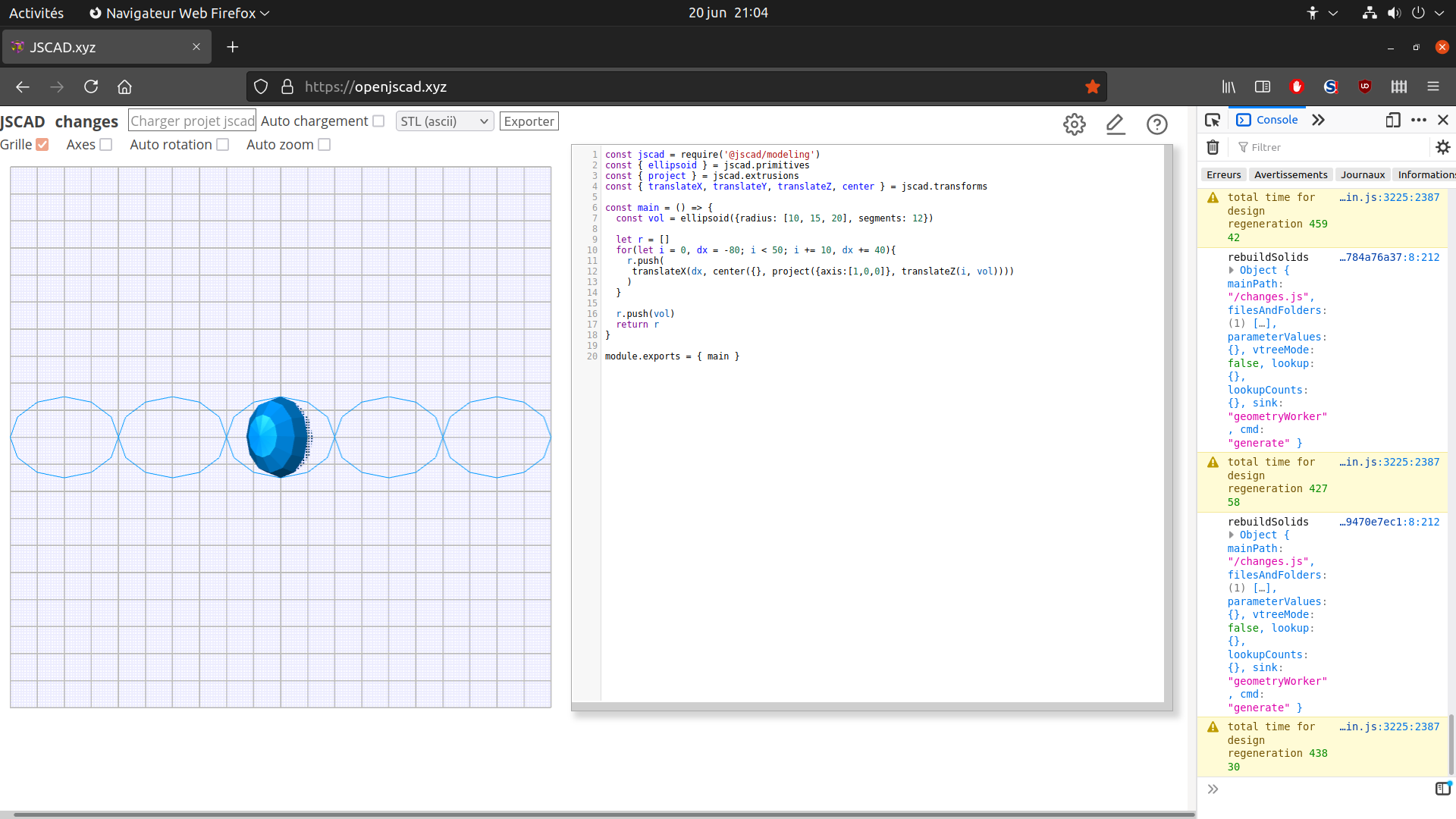# V2 feedback

• Hello, I'm updating my 2 axis slicer, replacing my functions by scission() and project(). And I'm having an odd error with project(). My volume is centered, and when I try to slice on the Y axis on some places that are <0 I have :

``````Error : the given points must define a closed geometry with three or more points
Line: 555"
``````

My test volume is symetric, and when I slice symetrically, it works. But what is odd is that at the left of the <0 part of the volume I can also slice. For the moment I will use my script with scission() and my pseudo-projection function vol2surf().

There's also another thing, when I use the script for some time, adding, moving and removing slices and also switching from 3d to 2d, the script hangs and I need to reload the page, sometimes, the script loads without the parameters, and I need to reload it again. There's maybe a memory issue, but I only have 8 Gb of RAM.

I'm also starting to create parametric furniture which is my main goal and was impossible to do as long as there were those T-junctions errors that are now overcome, and it looks promising. For the moment I'm exporting a furniture model to .STL, then import it when I want to slice it.

• @z3dev I think I get it now, it means that there's no simpler way to make the slices than what I already did at first.

• The WHOLE 3D shape is projected to 2D. If you want to project a ‘slice’ then use the booleans to make a ‘slice’, and project the ‘slice’.

• The origin and axis create a plane, which passes through the origin and lays facing up the axis. Just like the grid passes through [0,0,0] and faces up the Z axis.

The plane can be placed anywhere, which makes it easy to project different views of the 3D shape. No rotation or positioning of the 3D shape is required.

• @z3dev In order to simplify my process, I'm trying to directly use project to make the slices, and I'm beginning with an example, but I don't understand how to make several slices. First I used origin but it does not seem to work, then I'm translating the oldObject and it does not work, everytime I have the same slice.
here's my latest code :

``````const jscad = require('@jscad/modeling')
const { ellipsoid } = jscad.primitives
const { project } = jscad.extrusions
const { translateX, translateY, translateZ, center } = jscad.transforms

const main = () => {
const vol = ellipsoid({radius: [10, 15, 20], segments: 12})

let r = []
for(let i = 0, dx = -80; i < 50; i += 10, dx += 40){
r.push(
translateX(dx, center({}, project({axis:[1,0,0]}, translateZ(i, vol))))
)
}

r.push(vol)
return r
}

module.exports = { main }
``````

Maybe do I need to translate the object prior to the projection ?• @z3dev Yes I'm creating the slices for each axis from intersection of the model and a translated cuboid, then I create notches made from intersections of those slices, 1st axis slices have top half of those intersections subtracted and 2nd axis slices have bottom half, so they fit. Code is :

``````const main = (params) => {
const sc = 1, ep = params.ep *2

//const vv = require('./' + params.v + '.obj')
const vv = require('./' + params.v)

const vol = center({}, rotateX(degToRad(90), vv))
//const vol = center({}, vv)

let r = [], rH = [], rV = []
let bV = measureBoundingBox(vol)

// Recup parametres
const pv = Object.values(params)
const lH = pv.slice(0,10).filter(Number).map(x => (x-50)/100)
const lV = pv.slice(10,20).filter(Number).map(x => (x-50)/100)

// 1°) Traverses en X (H)
fH = bV - bV
mH = (bV + bV) / 2
var trH = cuboid( {
size: [ ep, 1+bV- bV, 1+bV - bV ]} )
for (let i = 0; i < lH.length; i++){
var t = intersect(vol, translateX(fH * lH[i], trH))
if (t.polygons.length > 0)
rH.push(t)
}

// 2°) Traverses en Y (V)
fV = bV - bV
mV = (bV + bV) / 2
var trV = cuboid( {
size: [ 1+ bV - bV, ep, 1+ bV - bV ]} )
for (let i = 0; i < lV.length; i++){
var t = intersect(vol, translateY(fV * lV[i], trV))
if (t.polygons.length > 0)
rV.push(t)
}

// 3°) Entrecroisement
var ur = union(intersect(union(rH), union(rV)));
var tmp = scission(ur)
console.log(tmp.length)
var eS = [], eH = [], eV = [];
for(let i=0; i< tmp.length; i++){
let p = tmp[i];
let b = measureBoundingBox(p),
//d = vec3.subtract(b, b);
d = [b-b, b-b, b-b];
let c1 = translate([b, b + ep/2, b],
cuboid({size: [d, d*2, d]}));
let c2 = translate([b + ep/2, b, b],
cuboid({size: [d*2, d, d]}));

eH.push(intersect(tmp[i], c1));
eV.push(intersect(tmp[i], c2));
}

rH = rH.map(x=> subtract(x, eV));
rV = rV.map(x=> subtract(x, eH));

if(params.mode == '3'){ // 3d
r.push(colorize([0,1,0,0.8], translateX(-70, subtract(vol, [rH,rV]))));

r.push(colorize([0.5,0.0,0], translateX(-70, rH.shift())));
if(rH.length > 0){
r.push(colorize([1,0,0], translateX(-70, rH)));
}

r.push(colorize([0,0,0.5], translateX(-70, rV.shift())));
if(rV.length > 0){
r.push(colorize([0,0,1], translateX(-70, rV)));
}
} else { // 2d
var dk = Math.max(fV, fH) *1.1
for(let ih = 0; ih < rH.length; ih++){
let b = measureBoundingBox(rH[ih]);
//r.push(translateX(dk *ih, union(vol2surf(rH[ih], 'x', b))))
r.push(translateX(dk *ih, union(project({axis:[1,0,0]}, rH[ih]))))
}
for(let iv = 0; iv < rV.length; iv++){
let b = measureBoundingBox(rV[iv]);
//r.push(translate([dk *iv, -dk - params.dky], union(vol2surf(rV[iv], 'y', b))))
r.push(translate([dk *iv, -dk - params.dky], union(project({axis:[0,1,0]}, rV[iv]))))
}
}

return r;
}
``````

Stack trace is maybe (I'm using Firefox) :

``````fromPoints blob:https://openjscad.xyz/333c3c92-e91a-42a7-8b4b-7dc2cdc255ce:555
map self-hosted:224
map self-hosted:224
main blob:https://openjscad.xyz/333c3c92-e91a-42a7-8b4b-7dc2cdc255ce line 38 > Function:137